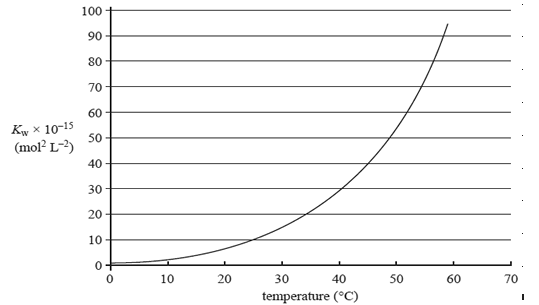Acid-base equilibrium chemistry 2009 VCE The value of the ionisation constant, Kw, of a sample of pure water at different temperatures is shown in the graph on the right. Which one of the following statements about the effect of increasing temperature on the pH and acidity of water is correct? A. The pH is always 7 and the water remains neutral. B. The pH decreases and the water remains neutral. C. The pH decreases and the water becomes acidic. D. The pH increases and the water remains neutral. SolutionIn a flask, 10.0 mL of a 0.100 M HCl solution is diluted to 1.00 L. In a second flask, 10.0 mL of a 0.100 M KOH solution is also diluted to 1.00 L. Which statement best describes the changes in pH in these flasks? A. pH change of the HCl solution increases by 2 pH change of the KOH solution decreases by 2 B. pH change of the HCl solution increases by 2, pH change of the KOH solution increases by 2. C. pH change of the HCl solution decreases by 2 pH change of the KOH solution increases by 2 D. pH change of the HCl solution decreases by 2 pH change of the KOH solution decreases by 2 SolutionThe NH4NO3 powder dissolves completely to form 300 mL of solution, with a pH of 5.04. i. Write an expression for the acidity constant, Ka, for the reaction between ammonium ions and water. Solution ii. Calculate the concentration, in mol L−1, of H3O+ ions in the 300 mL of solution. Solution iii. Calculate the mass, in grams, of NH4NO3 in the pack. Solution